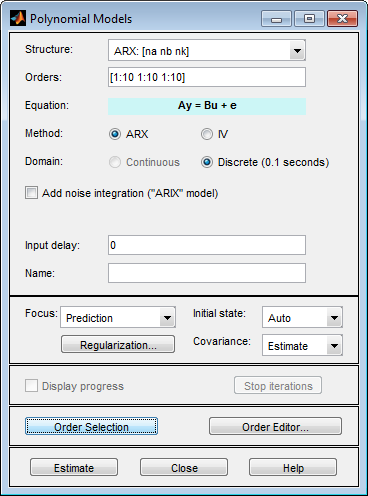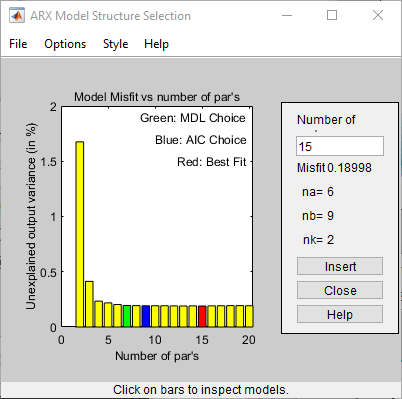## Preliminary Step – Estimating Model Orders and Input Delays

### Why Estimate Model Orders and Delays?

To estimate polynomial models, you must provide input delays and model orders. If you already have insight into the physics of your system, you can specify the number of poles and zeros.

In most cases, you do not know the model orders in advance. To get initial model orders and delays for your system, you can estimate several ARX models with a range of orders and delays and compare the performance of these models. You choose the model orders that correspond to the best model performance and use these orders as an initial guess for further modeling.

Because this estimation procedure uses the ARX model structure, which includes the A and B polynomials, you only get estimates for the na, nb, and nk parameters. However, you can use these results as initial guesses for the corresponding polynomial orders and input delays in other model structures, such as ARMAX, OE, and BJ.

If the estimated nk is too small, the leading nb coefficients are much smaller than their standard deviations. Conversely, if the estimated nk is too large, there is a significant correlation between the residuals and the input for lags that correspond to the missing B terms. For information about residual analysis plots, see topics on the Residual Analysis page.

### Estimating Orders and Delays in the App

The following procedure assumes that you have already imported your data into the app and performed any necessary preprocessing operations. For more information, see Represent Data.

To estimate model orders and input delays in the System Identification app:

1. In the System Identification app, select Estimate > Polynomial Models to open the Polynomials Models dialog box.

The ARX model is already selected by default in the Structure list.

Note

For time-series models, select the AR model structure.

2. Edit the Orders field to specify a range of poles, zeros, and delays. For example, enter the following values for na, nb, and nk:

`[1:10 1:10 1:10]`

Tip

As a shortcut for entering `1:10` for each required model order, click .3. Click to open the ARX Model Structure Selection window, which displays the model performance for each combination of model parameters. The following figure shows an example plot.4. Select a rectangle that represents the optimum parameter combination and click to estimates a model with these parameters. For information about using this plot, see Selecting Model Orders from the Best ARX Structure.

This action adds a new model to the Model Board in the System Identification app. The default name of the parametric model contains the model type and the number of poles, zeros, and delays. For example, `arx692` is an ARX model with na=6, nb=9, and a delay of two samples.

5. Click to close the ARX Model Structure Selection window.

Note

You cannot estimate model orders when using multi-output data.

After estimating model orders and delays, use these values as initial guesses for estimating other model structures, as described in Estimate Polynomial Models in the App.

### Estimating Model Orders at the Command Line

You can estimate model orders using the `struc`, `arxstruc`, and `selstruc` commands in combination.

If you are working with a multiple-output system, you must use the `struc`, `arxstruc`, and `selstruc` commands one output at a time. You must subreference the correct output channel in your estimation and validation data sets.

For each estimation, you use two independent data sets—an estimation data set and a validation data set. These independent data set can be from different experiments, or data subsets from a single experiment. For more information about subreferencing data, see Select Data Channels, I/O Data and Experiments in iddata Objects and Select I/O Channels and Data in idfrd Objects.

For an example of estimating model orders for a multiple-input system, see Estimating Delays in the Multiple-Input System in System Identification Toolbox Getting Started Guide.

#### struc

The `struc` command creates a matrix of possible model-order combinations for a specified range of na, nb, and nk values.

For example, the following command defines the range of model orders and delays `na=2:5`, `nb=1:5`, and `nk=1:5`:

`NN = struc(2:5,1:5,1:5))`

#### arxstruc

The `arxstruc` command takes the output from `struc`, estimates an ARX model for each model order, and compares the model output to the measured output. `arxstruc` returns the loss for each model, which is the normalized sum of squared prediction errors.

For example, the following command uses the range of specified orders `NN` to compute the loss function for single-input/single-output estimation data `data_e` and validation data `data_v`:

`V = arxstruc(data_e,data_v,NN);`

Each row in `NN` corresponds to one set of orders:

`[na nb nk]`

#### selstruc

The `selstruc` command takes the output from `arxstruc` and opens the ARX Model Structure Selection window to guide your choice of the model order with the best performance.

For example, to open the ARX Model Structure Selection window and interactively choose the optimum parameter combination, use the following command:

`selstruc(V);`

For more information about working with the ARX Model Structure Selection window, see Selecting Model Orders from the Best ARX Structure.

To find the structure that minimizes Akaike's Information Criterion, use the following command:

`nn = selstruc(V,'AIC');`

where `nn` contains the corresponding `na`, `nb`, and `nk` orders.

Similarly, to find the structure that minimizes the Rissanen's Minimum Description Length (MDL), use the following command:

`nn = selstruc(V,'MDL');`

To select the structure with the smallest loss function, use the following command:

`nn = selstruc(V,0);`

After estimating model orders and delays, use these values as initial guesses for estimating other model structures, as described in Using polyest to Estimate Polynomial Models.

### Estimating Delays at the Command Line

The `delayest` command estimates the time delay in a dynamic system by estimating a low-order, discrete-time ARX model and treating the delay as an unknown parameter.

By default, `delayest` assumes that na=nb=`2` and that there is a good signal-to-noise ratio, and uses this information to estimate nk.

To estimate the delay for a data set `data`, type the following at the prompt:

`delayest(data);`

If your data has a single input, MATLAB® computes a scalar value for the input delay—equal to the number of data samples. If your data has multiple inputs, MATLAB returns a vector, where each value is the delay for the corresponding input signal.

To compute the actual delay time, you must multiply the input delay by the sample time of the data.

You can also use the ARX Model Structure Selection window to estimate input delays and model order together, as described in Estimating Model Orders at the Command Line.

### Selecting Model Orders from the Best ARX Structure

You generate the ARX Model Structure Selection window for your data to select the best-fit model.

For a procedure on generating this plot in the System Identification app, see Estimating Orders and Delays in the App. To open this plot at the command line, see Estimating Model Orders at the Command Line.

The following figure shows a sample plot in the ARX Model Structure Selection window.

You use this plot to select the best-fit model.• The horizontal axis is the total number of parameters — na + nb.

• The vertical axis, called Unexplained output variance (in %), is the portion of the output not explained by the model—the ARX model prediction error for the number of parameters shown on the horizontal axis.

The prediction error is the sum of the squares of the differences between the validation data output and the model one-step-ahead predicted output.

• nk is the delay.

Three rectangles are highlighted on the plot in green, blue, and red. Each color indicates a type of best-fit criterion, as follows:

• Red — Best fit minimizes the sum of the squares of the difference between the validation data output and the model output. This rectangle indicates the overall best fit.

• Green — Best fit minimizes Rissanen MDL criterion.

• Blue — Best fit minimizes Akaike AIC criterion.

In the ARX Model Structure Selection window, click any bar to view the orders that give the best fit. The area on the right is dynamically updated to show the orders and delays that give the best fit.

## Related Examples